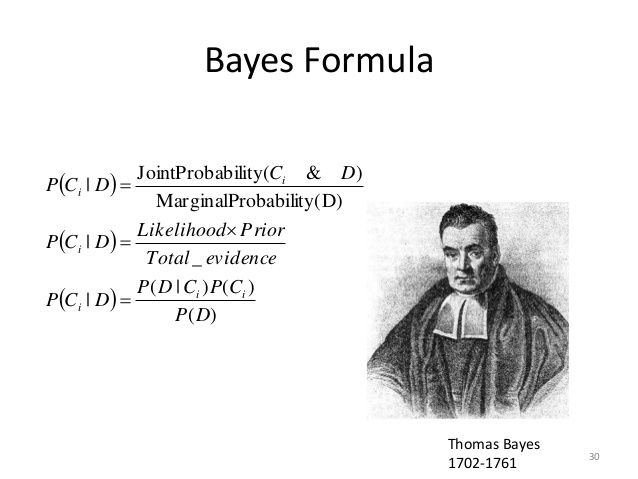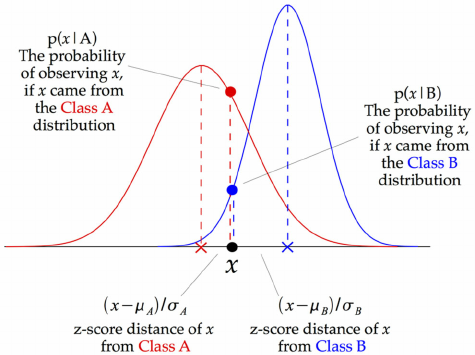# Naive Bayes

The Naive Bayes Classifier technique is based on the so-called Bayesian theorem and is particularly suited when the dimensionality of the inputs is high. Naive Bayes is a classi cation algorithm for binary and multiclass classi cation problems. The technique is easiest to understand when described using binary or categorical input values. It is called naive Bayes or idiot Bayes because of the calculation of the probabilities for each hypothesis are simpli ed to make their calculation tractable. Rather than attempting to calculate the values of each attribute value P(d1; d2; d3jh), they are assumed to be conditionally independent given the target value and calculated as P(d1jh) X P(d2jh) and so on. This is a very strong assumption that is most unlikely in real data, i.e. that the attributes do not interact.Naive Bayes can be extended to real-valued attributes, most commonly by assuming a Gaussian distribution. This extension of naive Bayes is called Gaussian Naive Bayes.References:

http://scikit-learn.org/stable/modules/naive_bayes.html